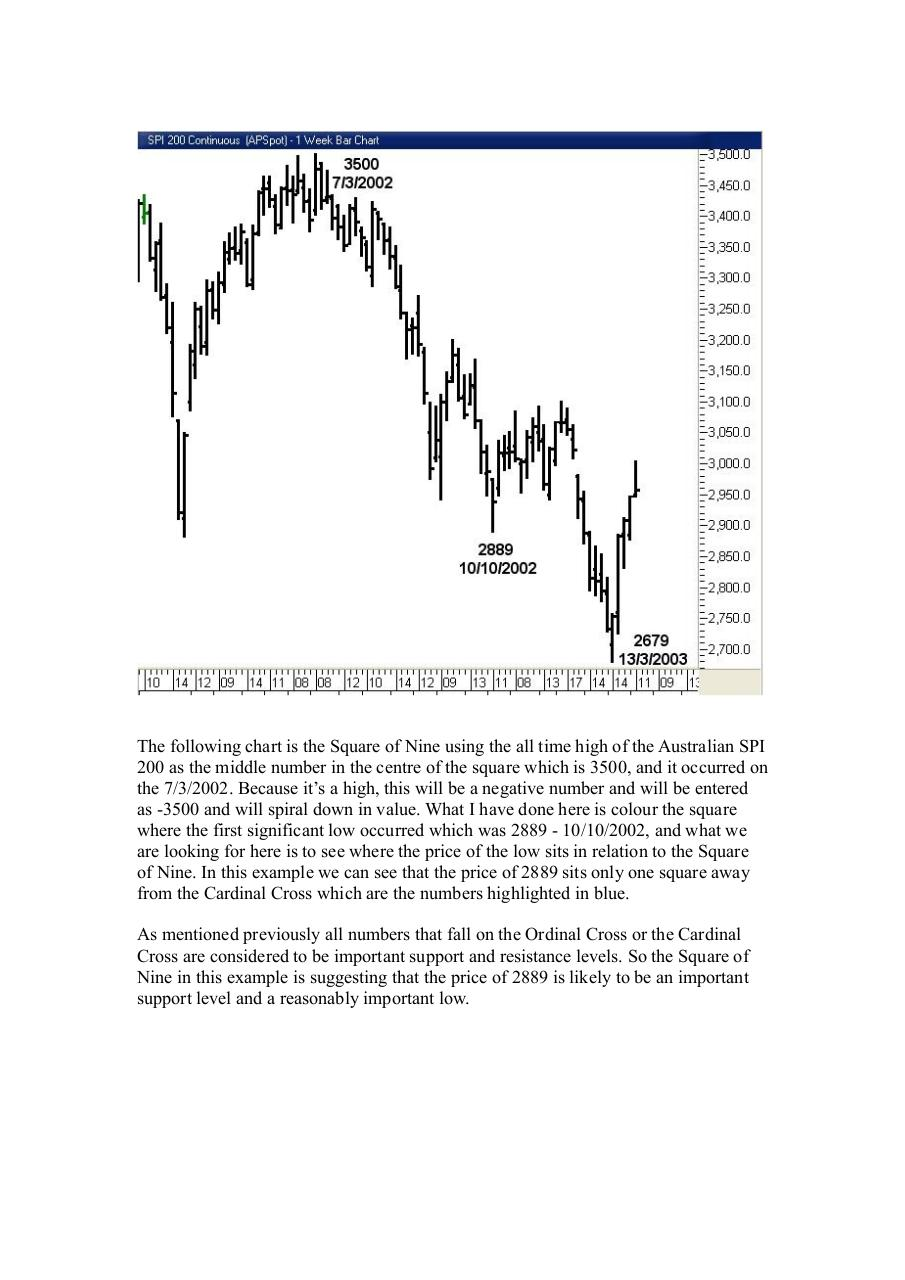# gann.pdfPage 1 2 3 4 5 6

#### Text preview

The following chart is the Square of Nine using the all time high of the Australian SPI
200 as the middle number in the centre of the square which is 3500, and it occurred on
the 7/3/2002. Because it’s a high, this will be a negative number and will be entered
as -3500 and will spiral down in value. What I have done here is colour the square
where the first significant low occurred which was 2889 - 10/10/2002, and what we
are looking for here is to see where the price of the low sits in relation to the Square
of Nine. In this example we can see that the price of 2889 sits only one square away
from the Cardinal Cross which are the numbers highlighted in blue.
As mentioned previously all numbers that fall on the Ordinal Cross or the Cardinal
Cross are considered to be important support and resistance levels. So the Square of
Nine in this example is suggesting that the price of 2889 is likely to be an important
support level and a reasonably important low.